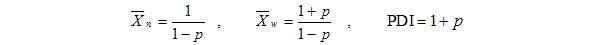# Background

## Stepwise Growth​

### Stepwise Growth Model​

In the stepwise growth sub-module, the dots will represent different monomer types. For instance, the red dots represent diols and the blue dots represent dicarboxylic acids used to make polyester. These dots are di-functional (they can bond to 2 other monomers). No actual “chemistry” is taken into account in the simulation aside from bonding rules. “Alcohol” type monomers are only able to react with “acid” type monomers. Monomers of the same type do not react with each other. The terms mono-ol and mono-acid indicate dots of alcohol (light red) and acid (light blue) types, respectively, which are mono-functional (can only bond with one other molecule). The terms mono-ol and mono-acid are not generally used chemical names, as they would simply be known as their chemical name such as ethanol or stearic acid. However, this nomenclature is utilized in the simulation to emphasize the monomer functionality. The crosslinker (green) dots represent tri-functional acids which are capable of forming crosslinks. However, in general, crosslinking agents can be of either acid or alcohol type and can have any functionality greater than two.

### Monomer Functionality and Gelation​

By adding mono-functional groups (which react to one other monomer), the user can investigate the end-capping effects of these groups as well as the end-capping effect of a stoichiometric imbalance of the monomers. Adding tri-functional groups (green dots), labeled “Crosslinker” in the simulation, in various concentrations can demonstrate molecular weight increase, branching, and gelation. This module serves an excellent tool for demonstrating gelation phenomena (see Examples), because when viewing the model in the mosaic format, it shows how the polymer instantly becomes one enormous molecule of infinite molecular weight. Gelation can be predicted to occur at a given conversion if the average functionality is greater than or equal to 2, assuming the monomers and crosslinkers are stoichiometricly balanced. In this case, $f$ is defined as the average functionality of all of the monomers. For instance, $f$ in the example problem would be defined as: : f = (700 x 2 + 400 x 2 + 200 x 3) / (700 + 400 + 200) = 2.15

Then, the critical conversion at which gelation will occur is defined as: :$P_c = \frac{1}{\sqrt{f-1}}$ which in this example equals 0.93. Unfortunately, this derivation only applies strictly for very large numbers of monomers in a 3-D network. The derivation also assumes that the first, second, and third bond will form with equal reactivity. Although the simulation does not explicitly enforce any reactivity for these bonds, each additional bond experiences a lower reactivity due to the steric hinderance of the existing bonds. Despite the three bad assumptions made in this equation, it works fairly well as an approximation in this simulation. The simulation is also fairly reliable for exhibiting gelation at high conversions as long as $f > 2$.

### Stepwise Growth Kinetics​

Assuming stoichiometric monomer concentrations of diols and diacids, the rate of polymerization is :$\frac{d[M]}{dt} = -k [M]^2$ where $[M]$ is the unreacted concentration of either monomer. Note the reaction constant, $k$, may be dependent on temperature. The conversion without catalysis is expected to be related to time via
:$\frac{1}{1-p} = [M_0]k t + 1.$ Note the conversion can be converted to the unreacted monomer concentration by multiplying the initial monomer concentration by $(1-p)$. The number-average degree of polymerization, the weight-average degree of polymerization, and for the polydispersity index should be: :The calculation of the maximum molecular weight for a nonstoichiometric mixture should simply be the total mass divided by the number of monomers in excess, which should approach infinity with perfect stoichiometry. The effects of temperature may also be modeled. Moderate temperature increases should increase the rate of reaction by increasing the number of molecular collisions. However, excessive heating should cause de-polymerization as the thermal forces rip the polymer apart. Note that the derivations of these equations can be found in G. Odian’s “Principles of Polymerization, 3rd ed.”

Various molecular weight distributions as initiator concentrations, monomer concentrations, initiator dissociation rates, chain transfer rates, and termination mechanisms can be investigated. The development and the resultant molecular weight distribution is significantly different from the step-wise growth sub-module. Having both of these sub-modules built into the same simulation will help students visualize how the different polymerization reactions function and how they result in significantly different polymers. Note that it is also possible to have cross-linking in free radical chain addition, although cross-linking groups are not included in this simulation.